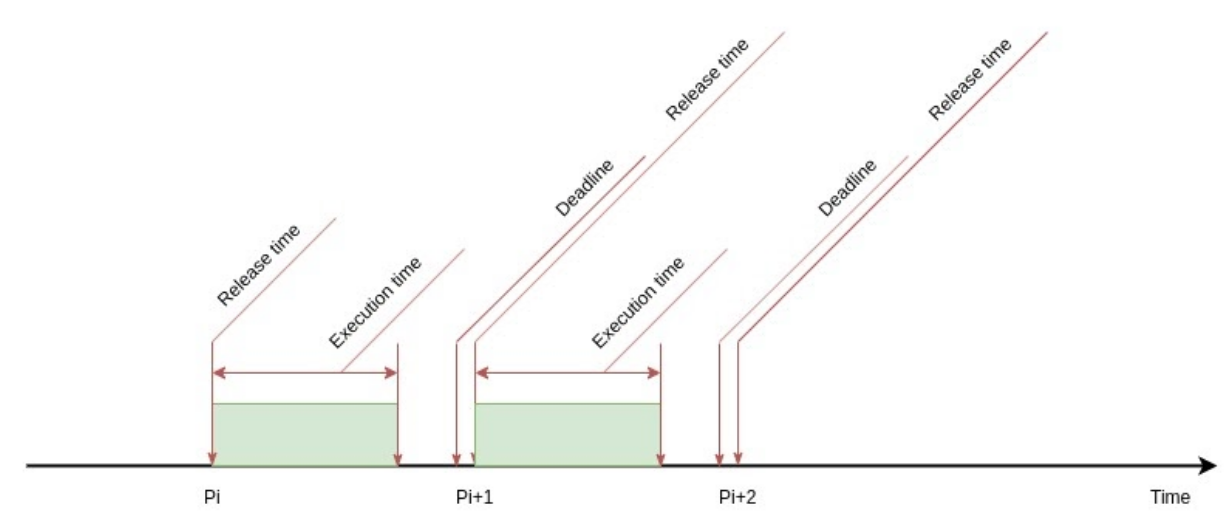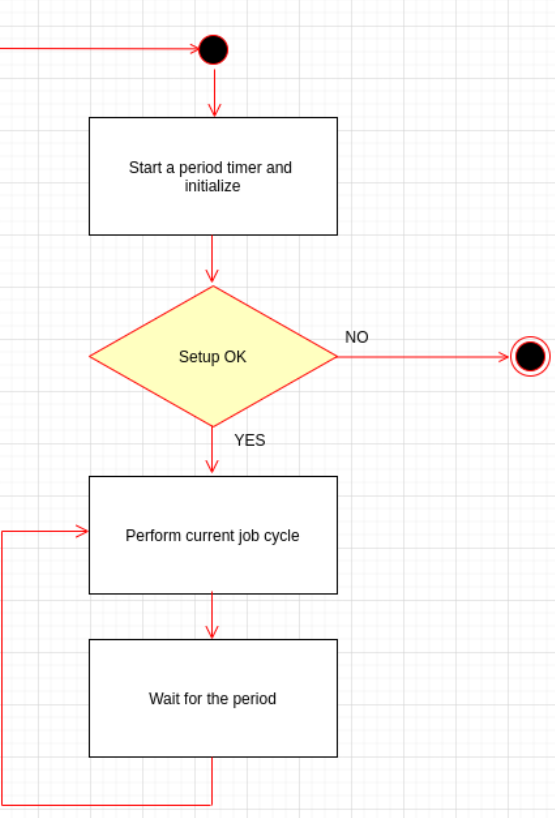# Implement Real-time Periodic Task for RTOS using Timers

Van C. Ngo · February 12, 2020

It is very common for real-time applications to have tasks that need to run periodically, for example to scan inputs or to generate regular outputs.

A naive solution is to use an infinite loop with a sleep at the end. However, the duration of period will vary as the execution time varies. One can claim that we can compute the different time between the execution time and period time for sleeping. But it is only true when the execution time is always less than the period. If the execution time is bigger than the period, then the period is not accurate. To create accurate periodic tasks we need to use timers. In this post, I will show how timers work in QNX, especially with regard to multi-threaded applications.

A real-time periodic task as depicted in the following figure, denoted by a tuple T = <P, E, D>, where:

P is the period of the task, e.g. the time interval between the release times of two consecutive jobs E is the execution time of the task D is the relative deadline of the task Relative deadline means the desired time duration counted from the beginning of the current cycle or period to the time of job completion. For the current period, given P and the absolute deadline d, the relative deadline D = d - P. In practice, we need to ensure that the (worst-case) execution time (by measuring) E is smaller than the relative deadline D in order to guarantee the hard real-time deadline properties.For example, consider a task with period P = 10ms, the (worst-case) execution time E = 3ms, and the relative deadline D = 9ms. Thus, the job of this task is released first at t = 0, then it executes for 8ms and then the next job is released at t1 = 10ms and so on. The jobs are released at t = 10k where k = 0, 1,...

## Implementation with Periodic Timer

In the example code, I have separated out the timer code into a separate class, called PeriodicTimer. The main functionalities are following:

1. bool setupTimer(const int sigNo): Set up a periodic timer with the given signal number emitted by the timer every period
2. bool startTimer(const timespec& period): Start the timer with the given period
3. void waitPeriod(): When the timer expires, the defined signal will be emitted, called pending signal. This function blocks the calling thread until the signal emitted and the pending signal is caught. For example, the timer period is 100ms, a thread calls this function at time point 80ms, so the thread is blocked until the defined signal emitted at time point 100ms. If the thread calls this function at time point 110ms, the function returns immediately, so the thread will not be blocked.
4. int overrunPeriods(): Compute the number of overrun periods in case the task execution time arrives after the specified period. So a timer expires while there is a pending signal that is not caught yet. This function returns the number of expirations. For example, the timer period is 100ms and a thread execution time is 210ms. So at time point 100ms, a defined signal is emitted (a pending signal). At time point 200ms, another signal is emitted, however, there is already a pending signal that is not caught yet. Therefore, at time point 210ms, the thread calls to waitPeriod(), the number of overrun period is 1.

#ifndef _PERIODIC_TIMER_H_
#define _PERIODIC_TIMER_H_

#include <iostream>
#include <time.h>
#include <signal.h>
#include <sys/siginfo.h>

/**
* \brief Timer signal information
*/
struct TimerSignal
{
int signalNumber{0};
sigset_t alarmSignalSet{};
};

/**
* \brief Defines a QNX POSIX timer
*/
class PeriodicTimer
{
public:
PeriodicTimer() = default;

virtual ~PeriodicTimer();

bool setupTimer(const int sigNo);

bool startTimer(const timespec& period);

bool deleteTimer();

void waitPeriod();

int overrunPeriods();

private:
bool setSignal(const int sigNo);

timer_t timerID_{};
bool isSetup_{false};
bool isStarted_{false};
timespec period_{0, 0};
TimerSignal timerSignal_{};
sigevent signalEvent_{};
};

#endif // _PERIODIC_TIMER_H_

#include "PeriodicTimer.h"

#include <time.h>
#include <signal.h>
#include <sys/siginfo.h>

PeriodicTimer::~PeriodicTimer()
{
deleteTimer();
}

bool PeriodicTimer::setupTimer(const int sigNo)
{
bool result{false};
if ((isSetup_ == false) && (setSignal(sigNo) == true))
{
// Create a timer that will generate the configured signal
signalEvent_.sigev_notify = SIGEV_SIGNAL;
signalEvent_.sigev_signo = timerSignal_.signalNumber;
signalEvent_.sigev_value.sival_ptr = reinterpret_cast<void*>(&timerID_);
result = (timer_create(CLOCK_REALTIME, &signalEvent_, &timerID_) == 0);
isSetup_ = result;
}
else
{
std::cout << "Timer has been already set up or invalid signal number" << std::endl;
}

return result;
}

bool PeriodicTimer::startTimer(const timespec& period)
{
bool result{false};
timespec zeroTime{0, 0};
const bool validPeriod = timeGreater(period, zeroTime);
if ((isStarted_ == false) && (isSetup_ == true) && (validPeriod == true))
{
struct itimerspec timerInterval
{
};
period_ = period;
// Make the timer periodic
timerInterval.it_interval = period_;
timerInterval.it_value = period_;
// Start the timer, flag 0 means relative time
result = (timer_settime(timerID_, 0, &timerInterval, nullptr) == 0);
isStarted_ = result;
}
else
{
std::cout << "Timer has been already started or is not set up" << std::endl;
}

return result;
}

bool PeriodicTimer::deleteTimer()
{
bool result{false};
if (timer_delete(timerID_) == 0)
{
isSetup_ = false;
isStarted_ = false;
result = true;
}

return result;
}

void PeriodicTimer::waitPeriod()
{
{
std::cout << "Failed to wait for the next period" << std::endl;
}
// Compute the number of timer overrun periods
auto overruns = overrunPeriods();
if (overruns > 0)
{
std::cout << "Timer overruns " << overruns << " periods" << std::endl;
}
}

int PeriodicTimer::overrunPeriods()
{
int overruns = timer_getoverrun(timerID_);
if (overruns == -1)
{
std::cout << "Failed to compute the number of overrun periods" << std::endl;
}

return overruns;
}

bool PeriodicTimer::setSignal(const int sigNo)
{
bool result{false};
// Check that the sigNo is in the range [SIGRTMIN, SIGRTMAX]
if ((sigNo >= SIGRTMIN) && (sigNo <= SIGRTMAX))
{
timerSignal_.signalNumber = sigNo;
if (sigemptyset(&timerSignal_.alarmSignalSet) == 0)
{
result = (sigaddset(&timerSignal_.alarmSignalSet, sigNo) == 0);
}
}

return result;
}


Assume that periodicTimer_ is an object of PeriodicTimer. You call setupTimer to set up a timer. At the start of the thread, you call startTimer() with the period as a timespec value and then call waitPeriod() when the cycle job execution is complete. To show you what I mean, here is an example of a thread with a pre-defined period:

// Set up a timer
periodicTimer_.setupTimer(timerSignalNumber);

// ...

// Start the periodic timer
if (periodicTimer_.startTimer(period) == true)
{
// Execute the job periodically
while (getExitFlag() == false)
{
// Perform the cycle job
// Wait for the next period
periodicTimer_.waitPeriod();
}
}
// Call cleanup job


The thread data-flow is depicted by the following figure.